## New Maths Curriculum: Year 2 Multiplication and Division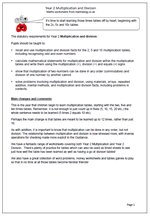Year 2 Multiplication and Division

It’s time to start learning those times tables off by heart; beginning with the 2x, 5x and 10x tables.
The statutory requirements for Year 2 Multiplication and division :

Pupils should be taught to:

• recall and use multiplication and division facts for the 2, 5 and 10 multiplication tables, including recognising odd and even numbers
• calculate mathematical statements for multiplication and division within the multiplication tables and write them using the multiplication (×), division (÷) and equals (=) signs
• show that multiplication of two numbers can be done in any order (commutative) and division of one number by another cannot
• solve problems involving multiplication and division, using materials, arrays, repeated addition, mental methods, and multiplication and division facts, including problems in contexts.

This is the year that children begin to learn multiplication tables, starting with the two, five and ten times tables. Remember, it is not enough to just count up in fives (5, 10, 15, 20 etc.) the whole sentence needs to be learned (5 times 2 equals 10 etc).
Perhaps the main change is that tables are meant to be learned up to 12 times, rather than just 10.

As with addition, it is important to know that multiplication can be done in any order, but not division. The relationship between multiplication and division is now stressed more, with inverse operations for checking made more explicit in the Guidance.

Year 2 multiplication and division statutory requirements

## New Maths Curriculum: Year 1 Multiplication and Division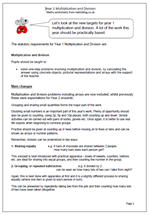Let’s look at the new targets for year 1 multiplication and division. A lot of the work this year should be practically based.

The statutory requirements for Year 1 Multiplication and Division are:

Multiplication and division

Pupils should be taught to:

•    solve one-step problems involving multiplication and division, by calculating the answer using concrete objects, pictorial representations and arrays with the support of the teacher.

Main changes

Multiplication and division problems including arrays are now included, whilst previously these were expectations for Year 2 onwards.

Grouping and sharing small quantities forms the major part of the work.

Doubling small numbers is an important part of this year’s work. Plenty of opportunity should also be given to counting, using 2p, 5p and 10p pieces, both counting up and down. Similar activities can be carried out with pairs of socks, gloves etc. Once again, it is better to use real life objects when beginning to combine groups.

Practice should be given at counting up in twos before moving on to fives or tens and can be shown as arrays or number patterns.
Remember, division can be understood in two ways:

1. Sharing equally               e.g. 6 bars of chocolate are shared between 2 people.
How many bars does each person get?

This concept is best introduced with practical apparatus – bowls of sweets, counters, buttons etc. are ideal for sharing into equal groups, and then counting the number in the group.

2. Grouping, or repeated subtraction                     e.g. 8 divided by 2
can be seen as how many lots of two can I take from eight?

Again, this is best done with apparatus at first and it is a slightly different process to sharing equally (where one item is given to each person in turn).

This can be answered by repeatedly taking two from the pile and then counting how many lots of two have been taken altogether.

Multiplication and division in Year 1

## Long multiplication: 3 by 2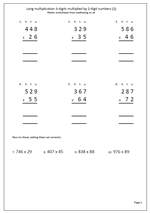I am often asked if there are more basic number sheets in the pipeline and so as to not disappoint people here is another page of long multiplication. The first six questions have been laid out in the correct way but the last four have been put in a horizontal position and will need laying out correctly.

The key with multiplying by 2-digit numbers is to multiply by the tens and then the units and finally add the two answers together. Some prefer to multiply by the units first: it makes no difference. Of course when multiplying by the tens digit it is important to remember to place a zero in the units column, ensuring that all other digits are then placed one place to the left and have a value ten times more.

It is also important to keep the numbers in straight columns to make adding up easier.

Long multiplication 3 by 2 (2)

## Resource of the Week: year 4 multiplication and addition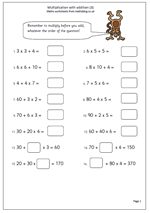This week we are highlighting an important part of year 4 maths which looks at the order of operations.

The rule is that multiplication should be worked out before the addition and in most of these questions that is fairly straightforward. However the questions towards the end are quite tricky.

Let’s look at 70 + ?? x 4 = 150

The best way to go about this is to go in reverse order; subtract 70 from 150 which leaves 80.

Then work out what number multiplied by 4 makes 80, which is 20.

Therefore the missing number is 20.

It is best to check this by going back to the original question; multiply 20 by 4 = 80 and then add 80 and 70 = 150, making the statement true.

Many children will find these very difficult to work out as they require several steps.

## Understanding multiplication in year 1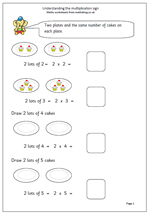At one time multiplication was not really introduced until year 2, but recent curriculum planning has included understanding multiplication into year 1, so here is a couple of pages to try to help with this.

At first multiplication can be seen as repeated addition so that:

2 added together 3 times is 2 + 2 + 2, or 3 lots of 2, or 3 times 2, or 3 x 2 (or 2 x 3).

The multiplication sign needs to be introduced, including using the words ‘times’, ‘multiply’ and ‘lots of’.

The second of the pages is quite a bit harder as there are no illustrations and various parts of the number sentences are missing. it would be a good idea to ‘talk through’ each number sentence, saying them out loud.

Understand the multiplication sign

## Short multiplication by 2, 5 and 10.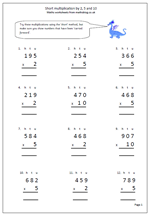When children are learning the standard methods of multiplication they need plenty of practice. With multiplication there are two distinct methods, the long and the short! Most schools tend to teach the long method first as it is clearer to show exactly what is happening, but it is also important for children to learn the short method, as we are looking to achieve fast accurate calculating.

This page keeps it simple by just multiplying by 2, 5 and 10; three tables that children should know ‘off by heart’.The questions have been laid out in the correct way so all that has to be done is:

a. Firstly to multiply by the units, remembering to carry any tens across to the tens column. These are usually placed under the answer.

b. Secondly multiply the tens remembering to carry any hundreds across to the hundreds column

c. Finally multiply the hundreds and write in the answer, including any thousands.

Short multiplication by 2, 5 and 10

## Short multiplication by 6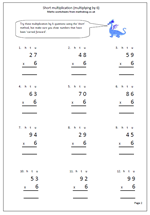Here is the latest worksheet which looks at the short multiplication method; this time multiplying 2-digit numbers by 6. It is called the short method because it eliminates the process of putting parts of the answer in two or three columns below, as in the ‘long’ method. The stages are the same as before:

1. writing the question out correctly so that it is in vertical format. it is a good idea to write a small h, t and u above to show the hundreds, tens and units columns.

2. Multiplying the units and carrying any of the tens across, below the answer line.

3. multiplying the tens and putting any hundreds into the answer.

More clarification of this method is shown on the worksheet.

The 6 times table is one of the harder tables to learn, but knowing it off by heart makes this type of multiplication so much easier.

Short multiplication by 6

## Short multiplication (multiplying by 3)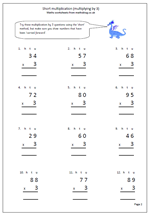Today I have published a page of multiplication questions which can be used to reinforce knowledge of the 3 times table as well as practising the short method of multiplication. it is called the short method because it does not involve writing to answer to each part of the question on a new line and then adding the totals, rather it is all done on one line. The main thing to remember is to carry the extra tens into the tens column and remember to add them into the final answer. A page at the start shows how to go about using this method.

The primary Framework for Mathematics is very keen to emphasise that written methods of multiplication should only be used when children have established a sound foundation of mental methods.

This page can be found in the Four Rules, Multiplication category, along with lots more.

Short multiplication for multiplying by 3

## More on multiplying by 2, 3, 5 and 10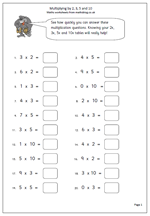I have had several requests for more pages for children to use who are beginning to learn the times tables, so here is another page which takes a quick look at multiplying by 2, 3, 5 and 10.

Children who know the times tables ‘off by heart’ will find this page easy and will fly through it in no time at all. Children who don’t know the times tables will find it much harder and will take much longer to complete it. This makes it an excellent quick short term assessment page.

There is no easy way to learn tables, just a lot of slog going over them again and again until they become known so well that they can be answered as quickly as answering your name. High expectations can push children to success and a times table (e.g. 3 times table) can be recited in full in less than 10 seconds, or even less than 7 seconds if it is really well known.

This page, and other similar pages, can be found in our Year 3 Calculating category, as well as in the four rulrs section of the site.

Multiplying by 2, 3, 5 and 10 (2)

## Maths worksheet: Multiplying by 2, 3, 5 and 10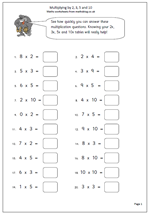Here is a straightforward maths worksheet which looks at multiplying by 2, 3, 5 and 10. Probably this page can be best used as an assessment page to see just how well these tables have been learned. They are the first four tables to be learnt and only the three times tables should cause much in the way of difficulty.

Some children do find it incredibly difficult to learn times tables so it is very worthwhile to keep coming back to them, repeating them time and time again until they become ‘second nature’.

When learning times tables it is important to say it in full and not just give the answers. For example a child might be able to recite counting on in twos: 2, 4, 6, 8, 10, 12 etc but if asked what six times two was would have to count up from two, probably using fingers to know when 6 has been reached. This is not an effective approach.

This page can be found in the Year 3 category on Calculating.